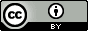## Wednesday, December 12, 2012

### Thermodynamics in solution: a brief guide for quantum chemists

2015.01.25: Please read this paper instead of this post. It turns out most of what I write here is wrong.

The thermodynamic properties such as enthalpy, entropy, and free energy you get from a vibrational analysis by programs such as GAMESS and Gaussian are those of an ideal gas at 1 bar pressure (and usually 298 K).  If you calculate free energy changes in solution (using methods such as PCM or COSMO) there are a few things you should do differently compared to gas phase calculations.  These things will only make a difference if you are computing free energy changes for processes where the number of particles change, such as binding free energies.  The corrections will cancel out for things such as conformational free energy differences.

Use Helmholtz free energies instead of Gibbs free energies
Experimental studies typically report Gibbs free energy changes ($\Delta G^\circ$), which are related to Helmholtz free energy changes ($\Delta A^\circ$) by$$\Delta G^\circ = \Delta A^\circ + p^\circ \Delta V$$ $\Delta V$ is the change in volume of the solution due to the reaction.  This volume change is negligible so $\Delta G^\circ = \Delta A^\circ$ is a good approximation.

The Gibbs free energy printed by quantum chemistry program correspond to an ideal gas where $pV=RT$ and the difference between $\Delta G^\circ$ and $\Delta A^\circ$ is much larger.

Computing free energies with semi-empirical methods
Most semiempirical methods such as AM1 and PM6 are parameterized such that the electronic energy matches experimental heat of formations ($\Delta H_f^\circ$) at 298 K.  So a gas phase free energy change should be computed as$$\Delta G^\circ=\Delta\Delta H_f^\circ-T\Delta S^\circ$$i.e. you don't need any of the enthalpy information printed out as part of the vibrational analysis.  A solution free energy change should be computed as $$\Delta A^\circ=\Delta\Delta U_f-T\Delta S^\circ$$where $\Delta U_f=\Delta H_f^\circ-RT$

Added 2013.08.16: However, dispersion and/or hydrogen bond corrected semi-empirical methods such as PM6-DH+ are parameterized against electronic binding energies.  So if you are computing binding free energies with such methods you need to add the translational, rotational, and vibrational enthalpy corrections to  $\Delta\Delta H_f^\circ$.

Change the standard state to 1 mol per liter
The translational entropy printed out depends on the volume of the system, which is computed as $V=RT/p$ = 24.79 liters.  For solution that volume should be 1 liter.  Most programs do not allow you to change volume so you must apply the correction to the entropy$$S_{soln}^\circ=S_{gas}^\circ+R\ln\left(\frac{1}{24.79}\right)$$ yourself

Is it OK to use the rigid rotor-harmonic oscillator approximation in solution?
Short answer: yes.  The derivations of the translational, rotational, and vibrational free energies are done for an isolated molecule in vacuum, while a molecule in solution interacts with solvent molecules.  However, a molecule in solution still has the exact same degrees of freedom as in the gas phase: it is free to explore the entire three dimensional volume and is free to adapt any rotational angle just like in the gas phase, so the resulting free energy expressions are the same.  Similarly, the individual molecules still have $3N-6$ internal vibrational frequencies.  The energy from the solute-solvent vibrations are included in the solvation free energy.

This blogpost was inspired by this excellent paper by Muddana and Gilson, and I thank Mike Gilson for helpful discussions.